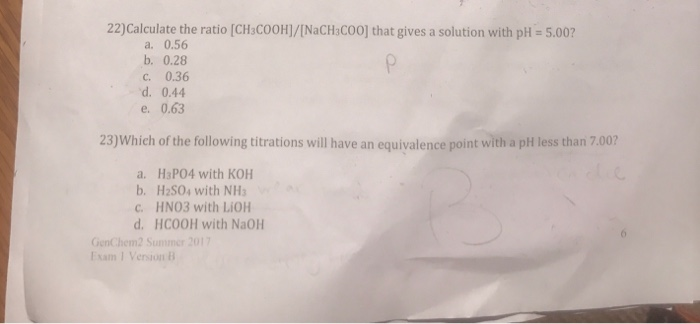# Question & Answer: Calculate the ratio [CH_3COOH]/[NaCH_3COO] that gives a solution with pH = 5.00? a. 0.56…..Calculate the ratio [CH_3COOH]/[NaCH_3COO] that gives a solution with pH = 5.00? a. 0.56 b. 0.28 c. 0.36 d. 0.44 e. 0.63 Which of the following titrations will have an equivalence point with a pH less than 7.00? a. H_3PO_4 with KOH b. H_2SO_4 with NH_3 c. HNO_3 with LiOH d. HCOOH with NaOH

First find out the acid dissociation constant from the data book

Ka = 1.7 *10^-5 for acetic acid
pKa = -log Ka
pKa = 4.77

Don't use plagiarized sources. Get Your Custom Essay on
Question & Answer: Calculate the ratio [CH_3COOH]/[NaCH_3COO] that gives a solution with pH = 5.00? a. 0.56…..
GET AN ESSAY WRITTEN FOR YOU FROM AS LOW AS \$13/PAGE

From Henderson–Hasselbalch equation
pH = pKa + log[ CH3COONa] / [CH3COOH]
5.00 = 4.77 + log[ CH3COONa] / [CH3COOH]
0.23 = log[ CH3COONa] / [CH3COOH]
[ CH3COONa] / [CH3COOH] =1.69
{CH3COOH}/{NaCH3COO} = 1 / 1.69
{CH3COOH}/{NaCH3COO} = 0.56